# Introduction to R Part 3 Algorithms Flowcharts Pseudocode

• Slides: 80
Download presentationIntroduction to R Part 3Algorithms Flowcharts Pseudocode Topics covered Variables Mathematical precedence Conditionals Chaining Loops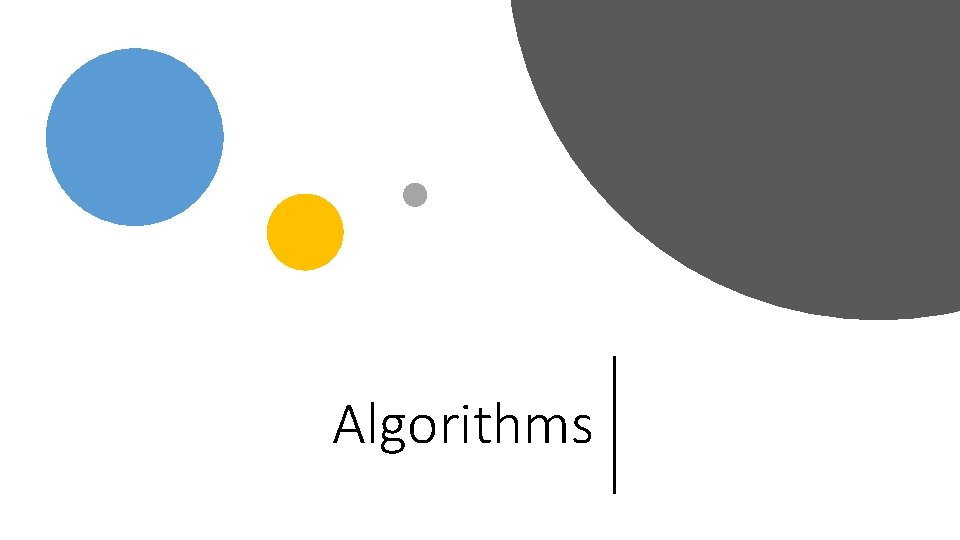AlgorithmsAn algorithm is not a computer programme Algorithms A piece of R code is not an algorithm A piece of R code is an implementation of an algorithm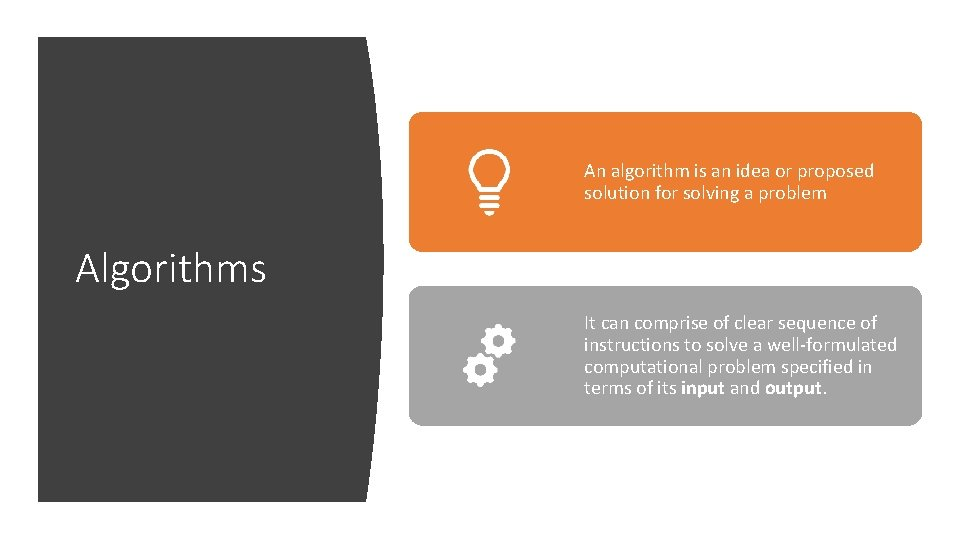An algorithm is an idea or proposed solution for solving a problem Algorithms It can comprise of clear sequence of instructions to solve a well-formulated computational problem specified in terms of its input and output.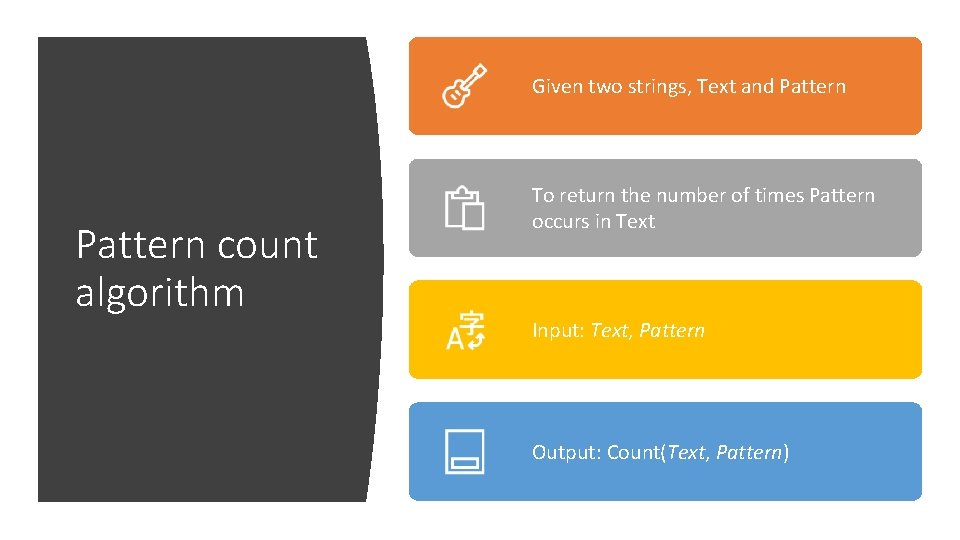Given two strings, Text and Pattern count algorithm To return the number of times Pattern occurs in Text Input: Text, Pattern Output: Count(Text, Pattern)Start from the first position of Text and check whether Pattern appears in Text starting at its first position. • If yes, draw a dot on a piece of paper. Move to the second position of Text and check whether Pattern appears in Text starting at its second position. • If yes, draw another dot on the same piece of paper. PATTERNCOUNT Continue until you reach the end of Text. Count the number of dots on the paper.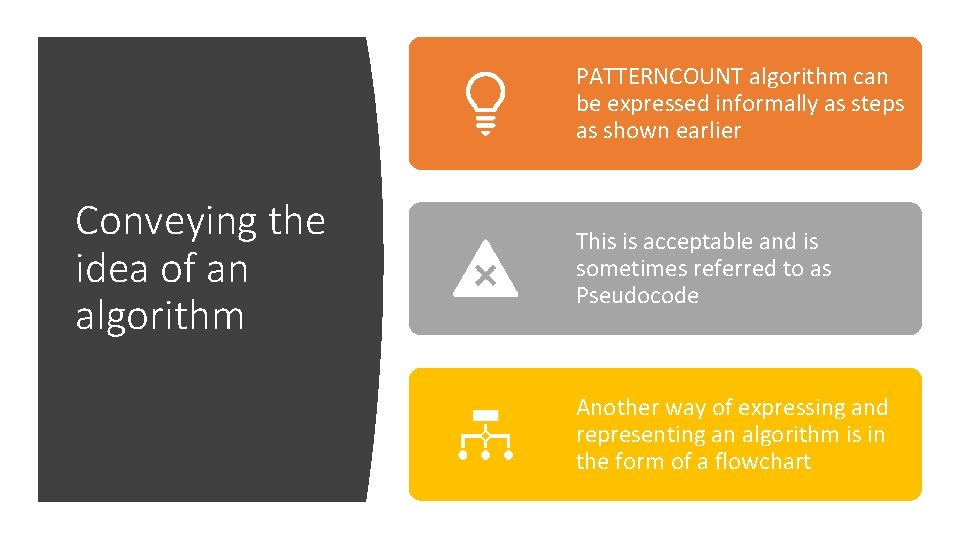PATTERNCOUNT algorithm can be expressed informally as steps as shown earlier Conveying the idea of an algorithm This is acceptable and is sometimes referred to as Pseudocode Another way of expressing and representing an algorithm is in the form of a flowchartPseudocode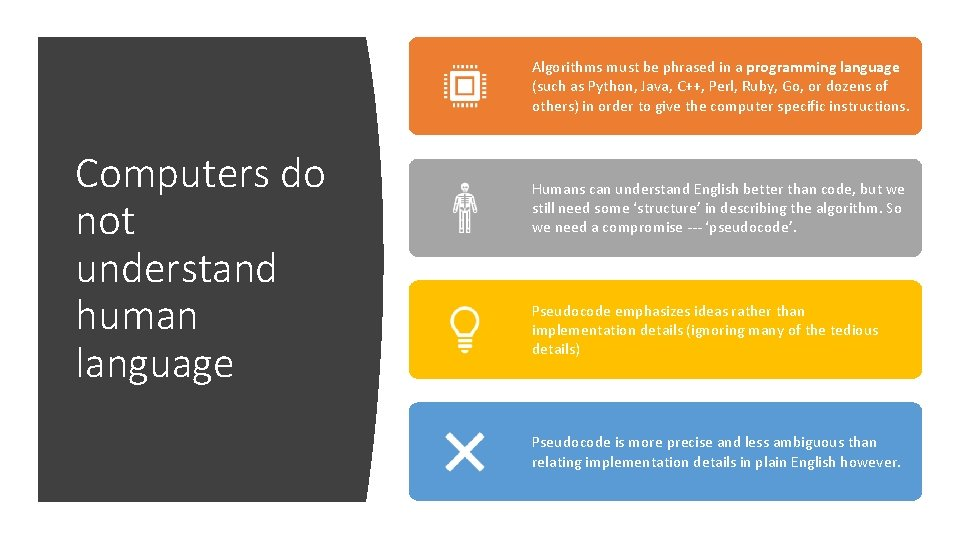Algorithms must be phrased in a programming language (such as Python, Java, C++, Perl, Ruby, Go, or dozens of others) in order to give the computer specific instructions. Computers do not understand human language Humans can understand English better than code, but we still need some ‘structure’ in describing the algorithm. So we need a compromise --- ‘pseudocode’. Pseudocode emphasizes ideas rather than implementation details (ignoring many of the tedious details) Pseudocode is more precise and less ambiguous than relating implementation details in plain English however.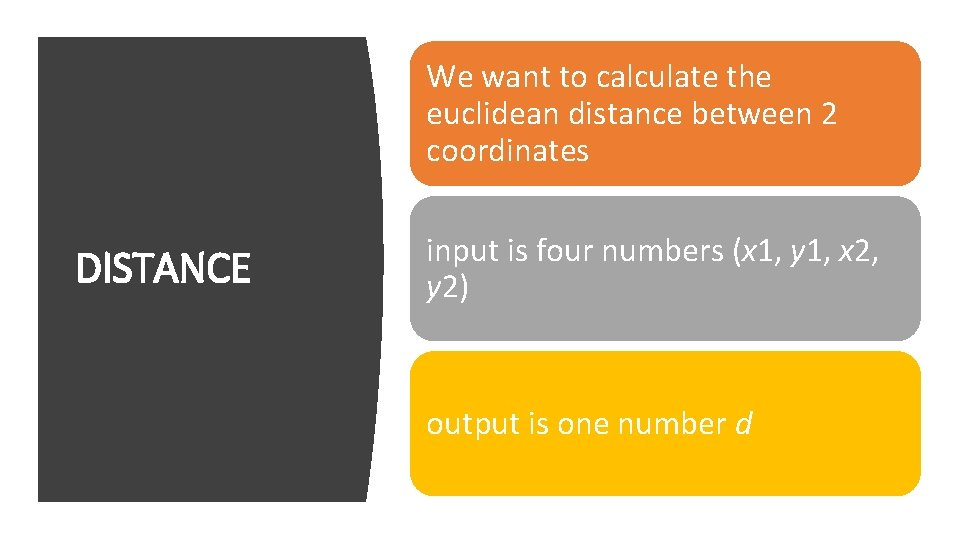We want to calculate the euclidean distance between 2 coordinates DISTANCE input is four numbers (x 1, y 1, x 2, y 2) output is one number d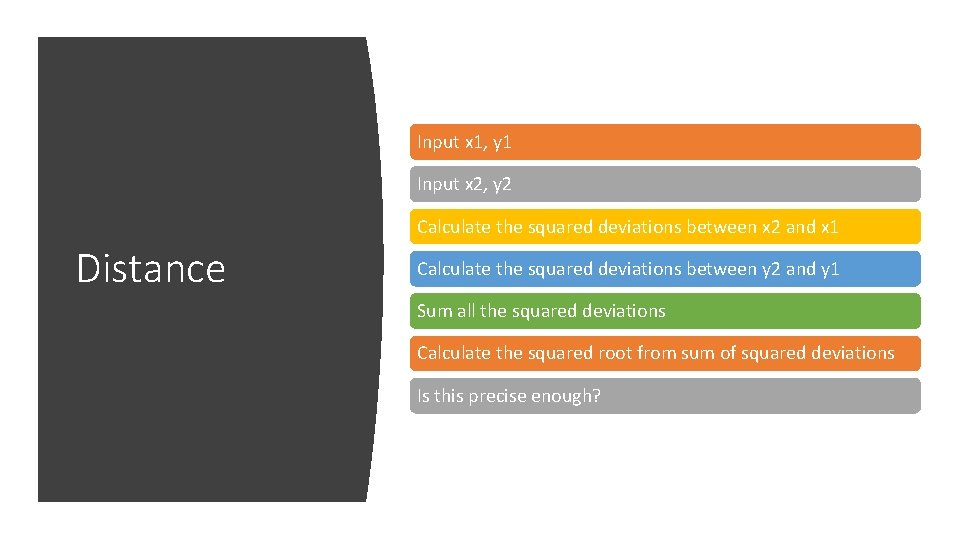Input x 1, y 1 Input x 2, y 2 Calculate the squared deviations between x 2 and x 1 Distance Calculate the squared deviations between y 2 and y 1 Sum all the squared deviations Calculate the squared root from sum of squared deviations Is this precise enough?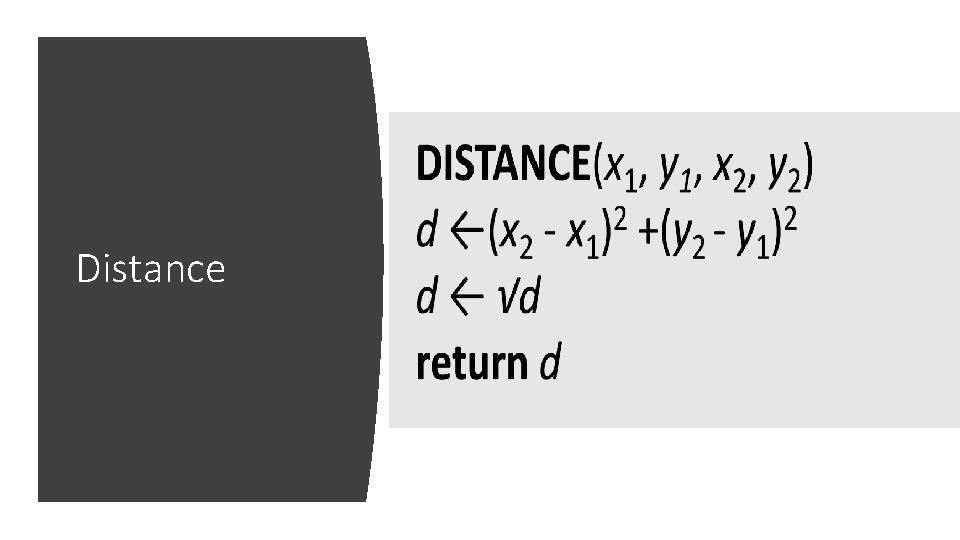Input x 1, y 1 Input x 2, y 2 Calculate the squared deviations between x 2 and x 1 Distance Calculate the squared deviations between y 2 and y 1 Sum all the squared deviations Calculate the squared root from sum of squared deviations Is this precise enough?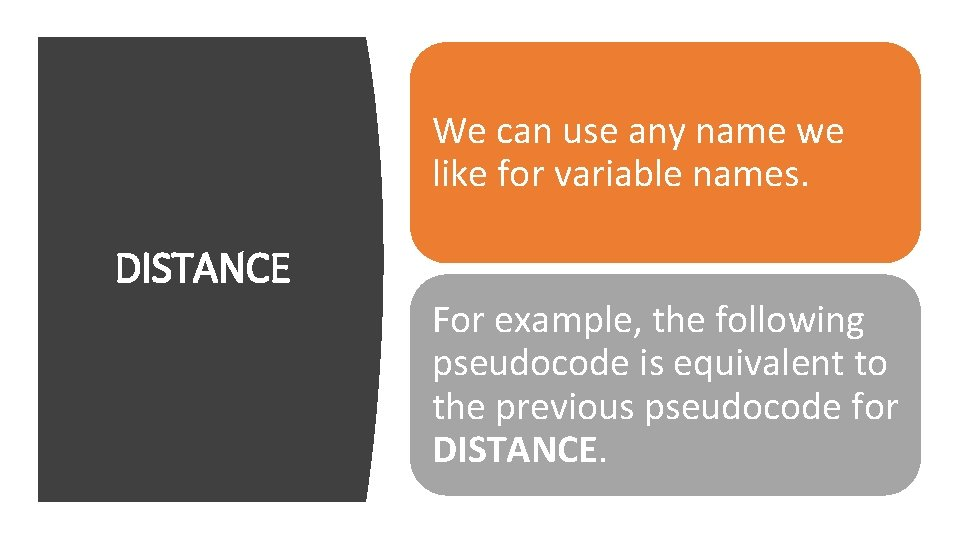We can use any name we like for variable names. DISTANCE For example, the following pseudocode is equivalent to the previous pseudocode for DISTANCE.Input x 1, y 1 Distance Input x 2, y 2 Calculate the squared deviations between x 2 and x 1 Calculate the squared deviations between y 2 and y 1 Sum all the squared deviations Calculate the squared root from sum of squared deviations Is this precise enough?Computer scientists are accustomed to pseudocode, biologists might decide that pseudocode is too cryptic and therefore useless Biologists and Pseudocode Modern biologists deal with algorithms on a daily basis, the language they use to describe an algorithm may be closer to a series of steps described in plain English. Some bioinformatics books are written without pseudocode. Unfortunately, this language is insufficient to describe the complex algorithmic ideas behind various bioinformatics tools that biologists use every day.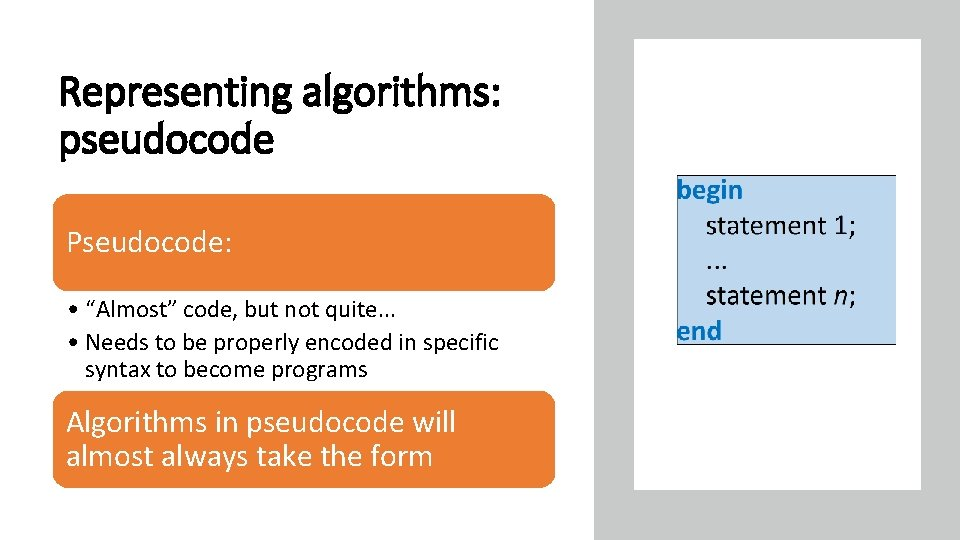Representing algorithms: pseudocode Pseudocode: • “Almost” code, but not quite. . . • Needs to be properly encoded in specific syntax to become programs Algorithms in pseudocode will almost always take the form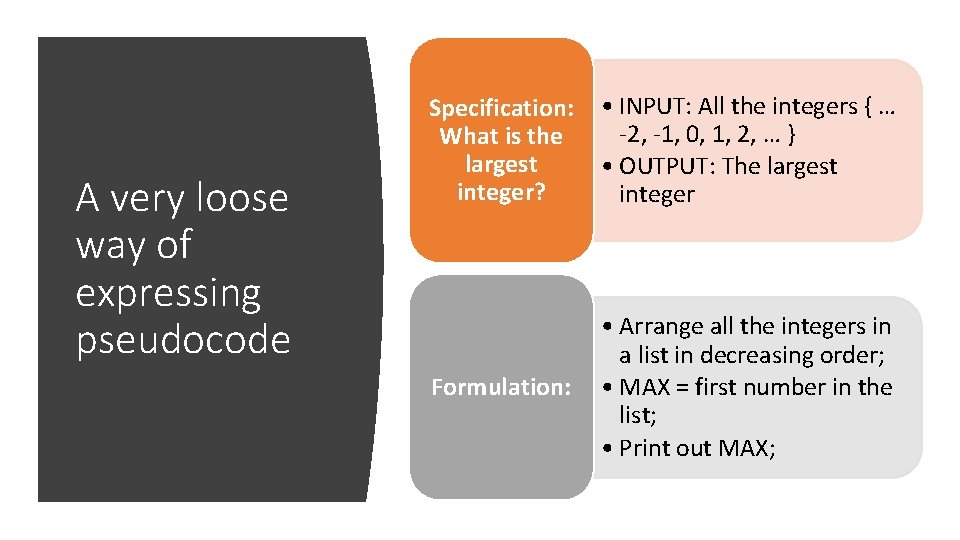A very loose way of expressing pseudocode Specification: What is the largest integer? • INPUT: All the integers { … -2, -1, 0, 1, 2, … } • OUTPUT: The largest integer Formulation: • Arrange all the integers in a list in decreasing order; • MAX = first number in the list; • Print out MAX;A more specific way of expressing pseudocodeFlowcharts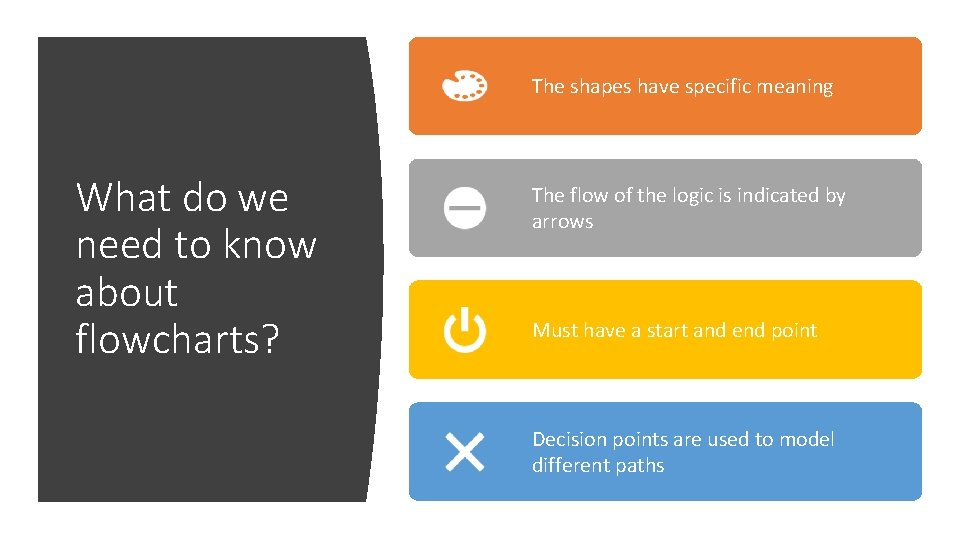The shapes have specific meaning What do we need to know about flowcharts? The flow of the logic is indicated by arrows Must have a start and end point Decision points are used to model different paths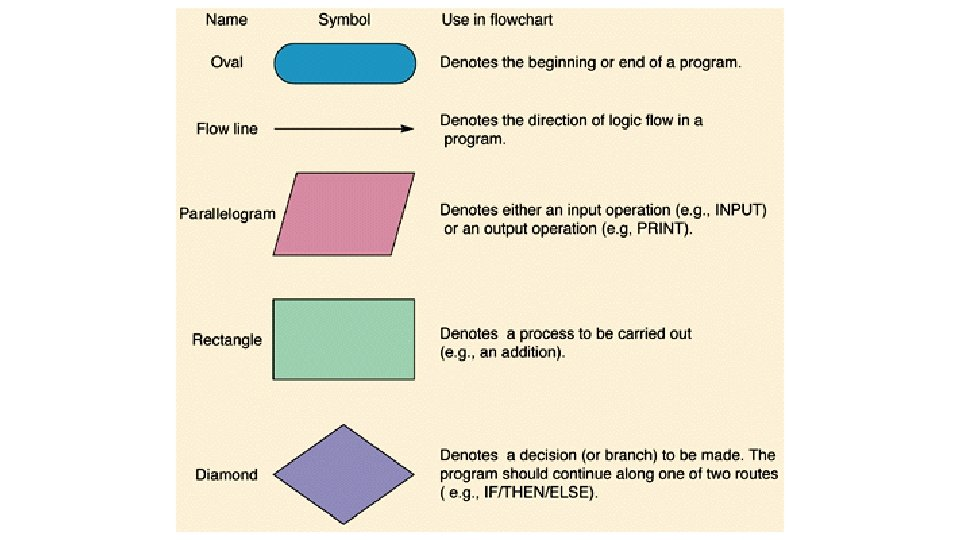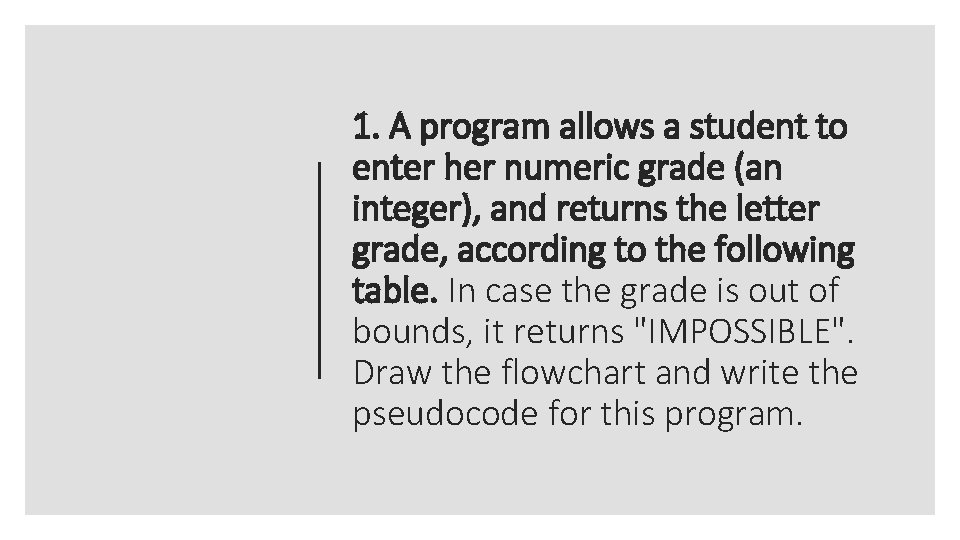1. A program allows a student to enter her numeric grade (an integer), and returns the letter grade, according to the following table. In case the grade is out of bounds, it returns "IMPOSSIBLE". Draw the flowchart and write the pseudocode for this program.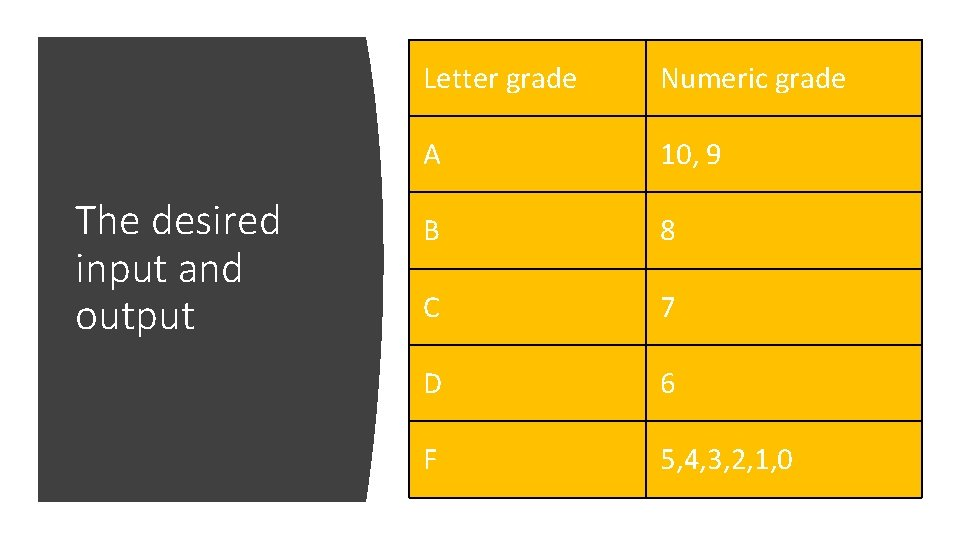The desired input and output Letter grade Numeric grade A 10, 9 B 8 C 7 D 6 F 5, 4, 3, 2, 1, 0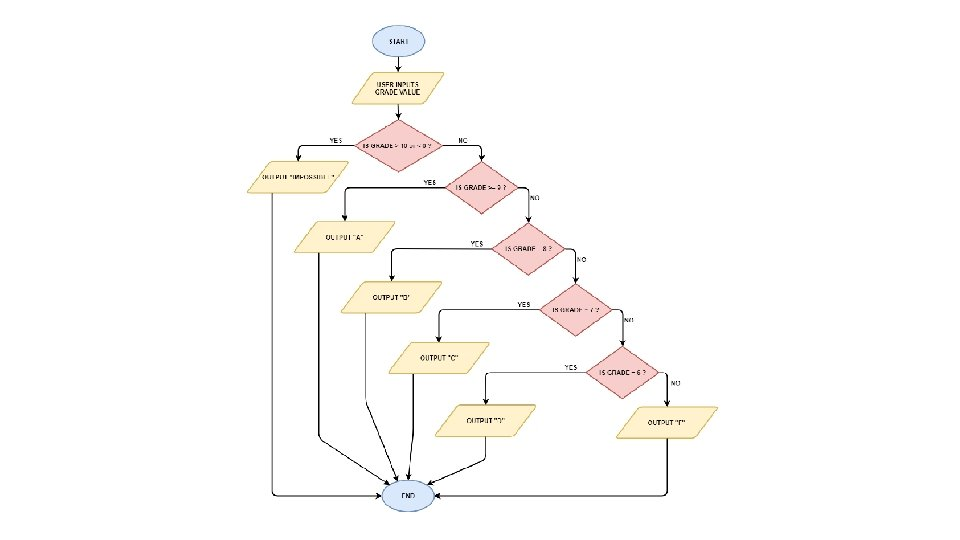Algorithm, pseudocode and program Algorithm • An algorithm is conceptual. Pseudocode • A pseudocode is written in plain English to express the algorithm • Human readable but less exact Program • Conversion of the pseudocode or algorithm into the formal instructions (syntax) used in a programming language • Machine readable instruction (very exact)VariablesIn statistics, a variable is a measurement of some quantifiable attribute. For example, height and weight are examples of variables. In computer science, a variable is a container that can be filled with data. Variables • A variable must be created (or instantiated) first When a variable has no data, it is empty • E. g. the expression ‘A = ()’ creates an empty variable A with nothing in it Filling up the container (variable) with some data requires a process called ‘assignment’ • E. g. the expression ‘A = 5’ means I assign the value of 5 to AVariables • Variables can take many forms • As a string/character type • e. g. “alice”, “paul”, “wilson” • As a numeric • e. g. 10, 1000 • As binary • 1 or 0 • As logical/boolean • True or FalseVariables • Variables can be made accessible to any part of the program (global) • Variables are only made accessible to a fixed part of the program (local) • P. S. A function is an autonomous segment of code that performs a specific role; its internal processes are kept separate from the rest of the program (we will get to that later) • Discussion for class: Why do you think it is necessary to keep some variables limited to only parts of the programme?Variables (in action) Instantiation A = () Assignment A=5 Reassignment A = 10 Global and local variable (Is the value of A 5 or 10? ) A=5 test = function(A) { A = 10 }Normally when we assign a value to a variable, we use ‘=‘ R also has a special assignment symbol In R, we may also use ‘<-’ in place of ‘=‘. So A <- 5 is the same as saying A = 5 Try writing this in your R console: A <- 5 A A=5 AA number (numeric) A word (string) Variable types Binary (basically 1 and 0) Logical ( True or False)There are 2 kinds of numeric variables --Integer and numeric (decimal/float) By default, R considers all numerical input as “numeric”. Numeric To know whether the variable is of numeric type, you use the is. integer() function. Try the following code: • • A <- 40 A is. integer(A) #This evaluates to False is. numeric(A) #This evaluates to True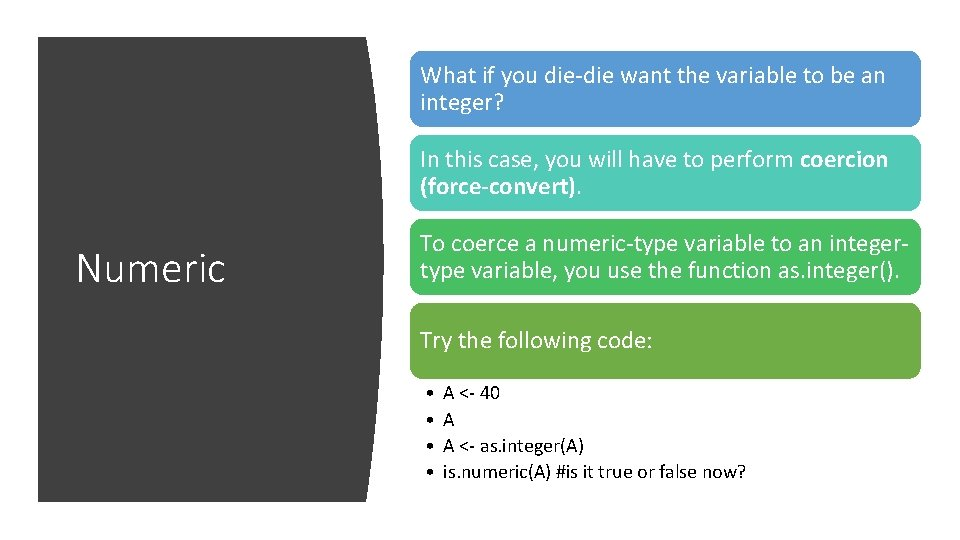What if you die-die want the variable to be an integer? In this case, you will have to perform coercion (force-convert). Numeric To coerce a numeric-type variable to an integertype variable, you use the function as. integer(). Try the following code: • • A <- 40 A A <- as. integer(A) is. numeric(A) #is it true or false now?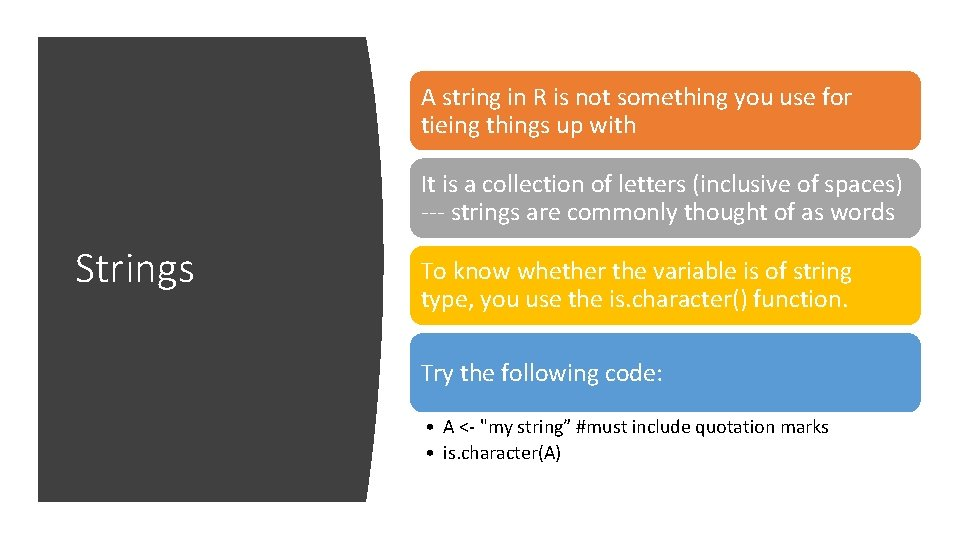A string in R is not something you use for tieing things up with It is a collection of letters (inclusive of spaces) --- strings are commonly thought of as words Strings To know whether the variable is of string type, you use the is. character() function. Try the following code: • A <- "my string” #must include quotation marks • is. character(A)Strings can also comprise of numbers. However, when a number is a string-type, no arithmetics can be performed on it. String To coerce a numeric-type to string-type, you use as. character(). Try the following code: • A <- 40 • is. numeric(A) • A <- as. character(A) • is. numeric(A) • is. character(A) • A #notice the double quotesYou normally think of binary as simply 1 s and 0 s But binaries are more than that, it is also a numbering system and also for encoding data in computers Binary For example: Hexadecimal code Although binary is an important variable type, it is not commonly dealt with in statistical computing, and R does not provide standard methods to deal with binary And so we will move on.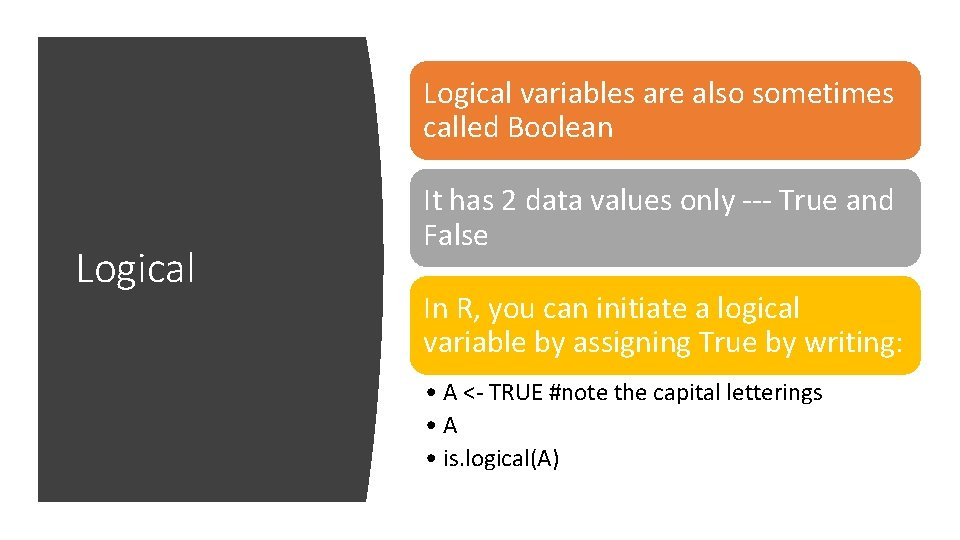Logical variables are also sometimes called Boolean Logical It has 2 data values only --- True and False In R, you can initiate a logical variable by assigning True by writing: • A <- TRUE #note the capital letterings • A • is. logical(A)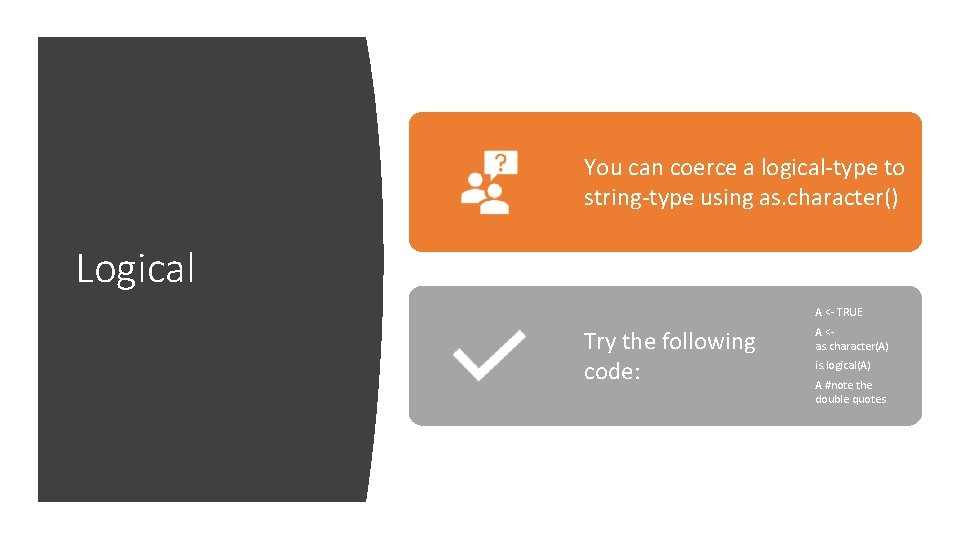You can coerce a logical-type to string-type using as. character() Logical A <- TRUE Try the following code: ABesides specifying the TRUE/FALSE directly, you may notice that you are already getting TRUE or FALSE messages when you are using functions like is. character() Logical You get a logical-type when evaluating a statement. • A <- 40 #a numeric • is. numeric(A) #TRUE • is. character(A) #FALSE Evaluating statements in programming will always result in a logical-type being returned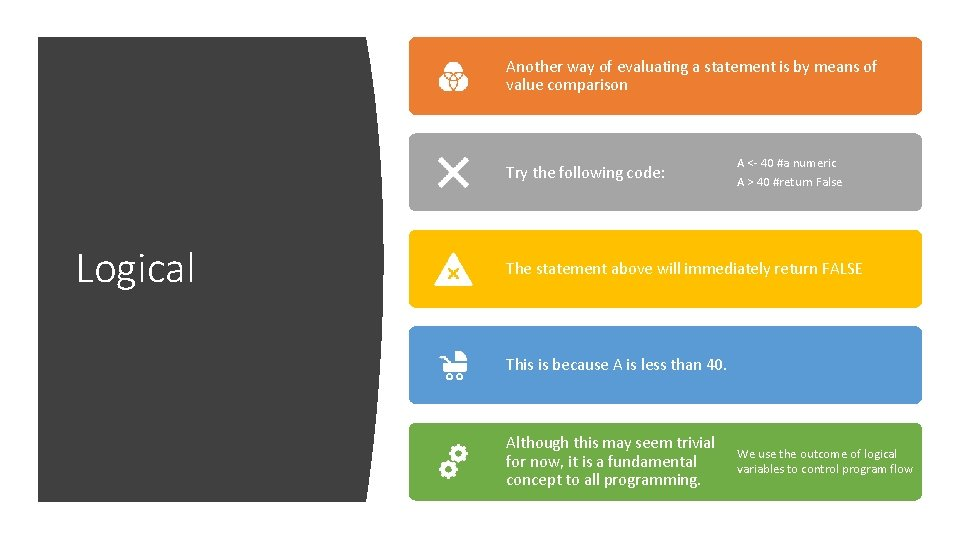Another way of evaluating a statement is by means of value comparison Try the following code: Logical A <- 40 #a numeric A > 40 #return False The statement above will immediately return FALSE This is because A is less than 40. Although this may seem trivial for now, it is a fundamental concept to all programming. We use the outcome of logical variables to control program flow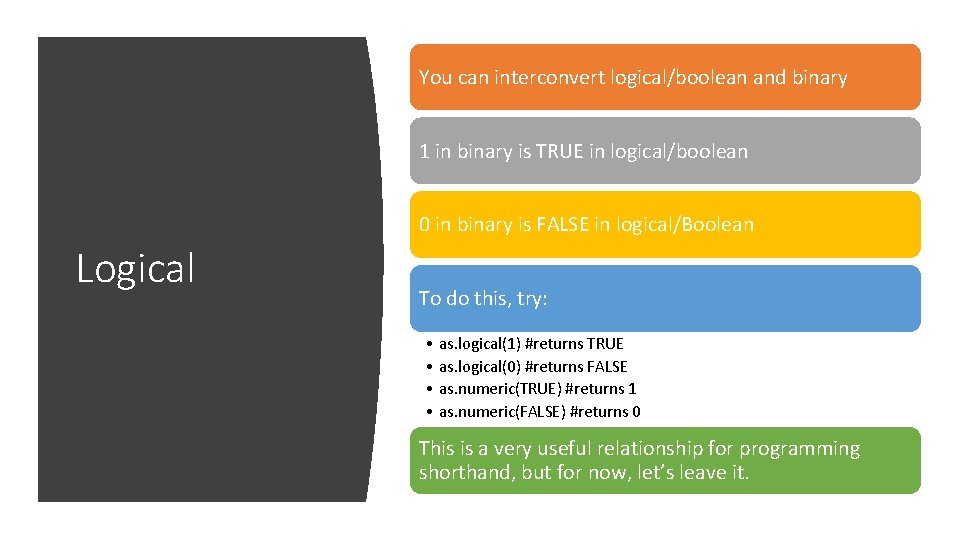You can interconvert logical/boolean and binary 1 in binary is TRUE in logical/boolean 0 in binary is FALSE in logical/Boolean Logical To do this, try: • as. logical(1) #returns TRUE • as. logical(0) #returns FALSE • as. numeric(TRUE) #returns 1 • as. numeric(FALSE) #returns 0 This is a very useful relationship for programming shorthand, but for now, let’s leave it.Mathematical precedence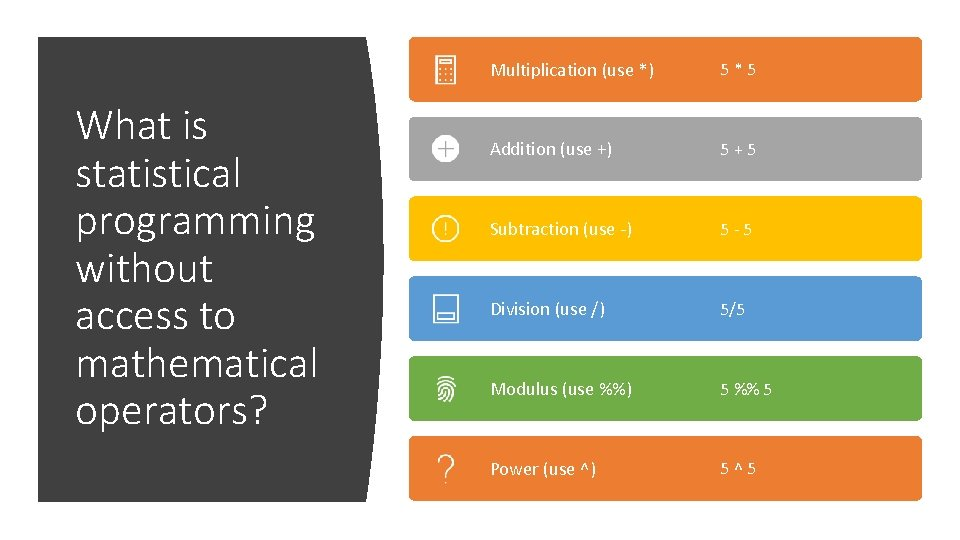What is statistical programming without access to mathematical operators? Multiplication (use *) 5*5 Addition (use +) 5+5 Subtraction (use -) 5 -5 Division (use /) 5/5 Modulus (use %%) 5 %% 5 Power (use ^) 5^5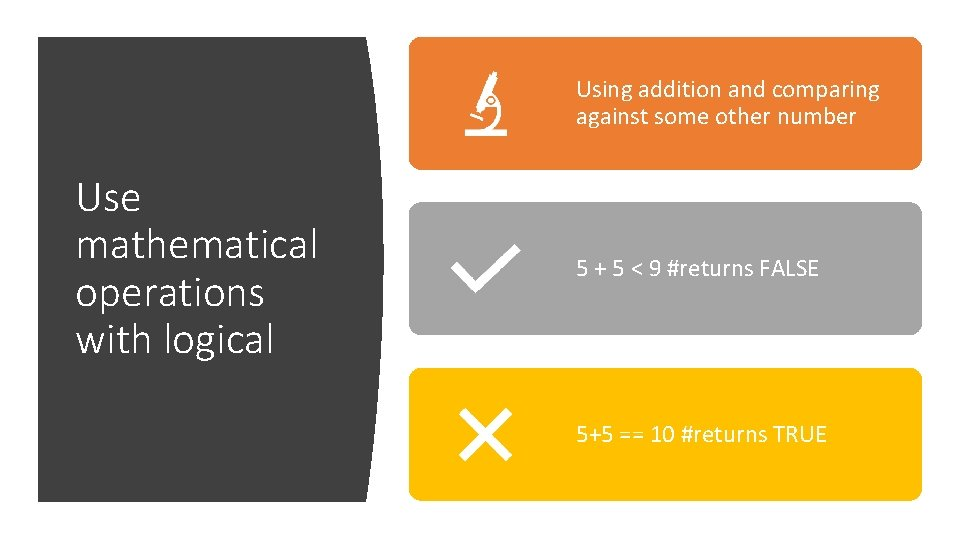Using addition and comparing against some other number Use mathematical operations with logical 5 + 5 < 9 #returns FALSE 5+5 == 10 #returns TRUE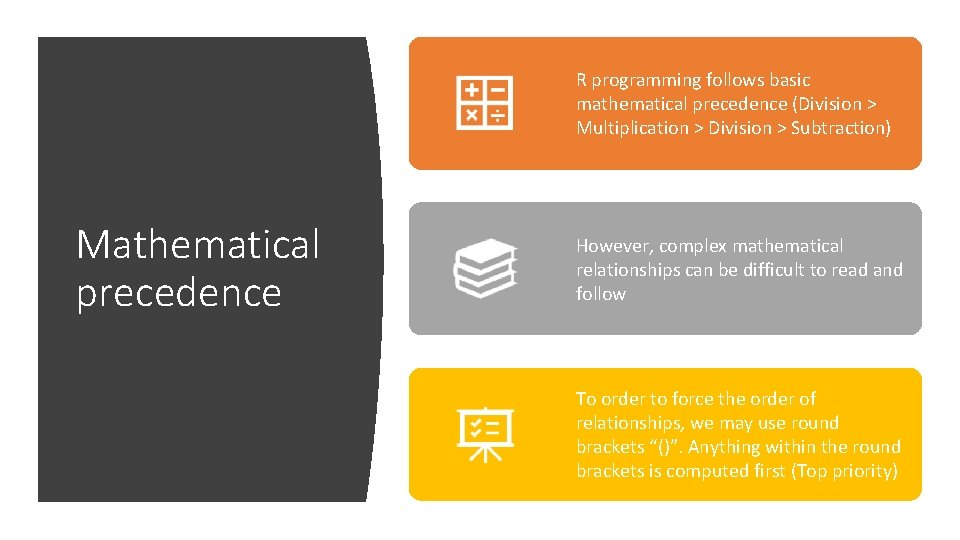R programming follows basic mathematical precedence (Division > Multiplication > Division > Subtraction) Mathematical precedence However, complex mathematical relationships can be difficult to read and follow To order to force the order of relationships, we may use round brackets “()”. Anything within the round brackets is computed first (Top priority)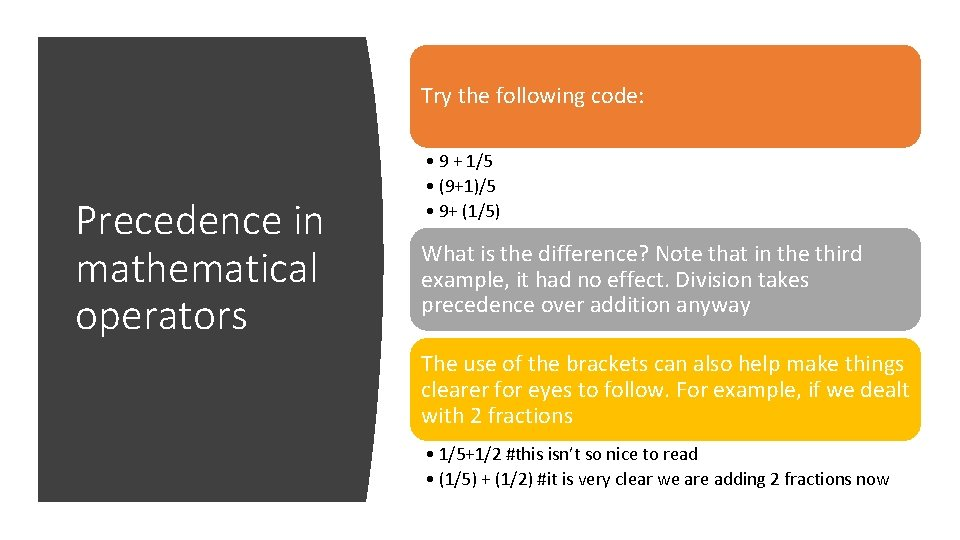Try the following code: Precedence in mathematical operators • 9 + 1/5 • (9+1)/5 • 9+ (1/5) What is the difference? Note that in the third example, it had no effect. Division takes precedence over addition anyway The use of the brackets can also help make things clearer for eyes to follow. For example, if we dealt with 2 fractions • 1/5+1/2 #this isn’t so nice to read • (1/5) + (1/2) #it is very clear we are adding 2 fractions nowMathematical precedence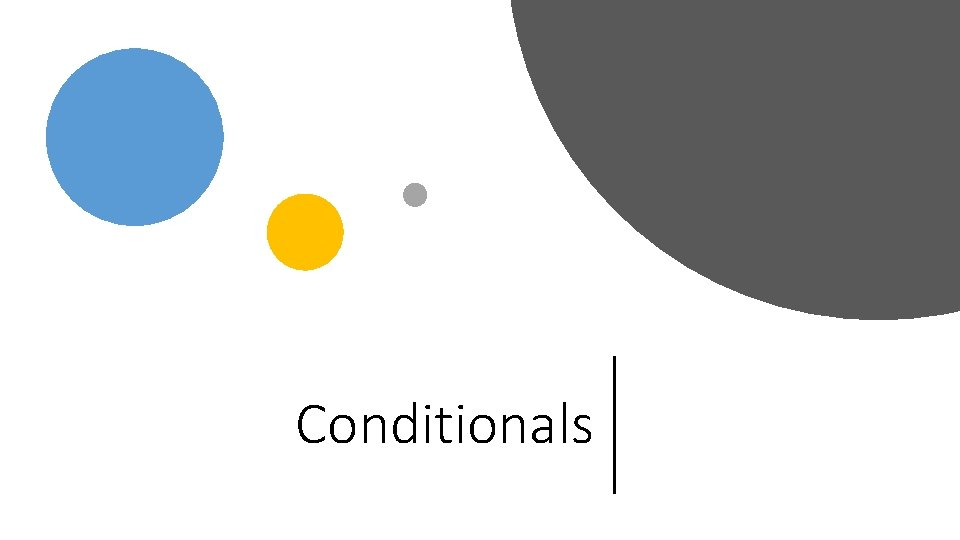ConditionalsAn conditional operation evaluates a line of code to see if it meets some condition Conditionals • If the condition is met, then it is true. • If the condition is not met, then it is false Common examples of operators: ‘if’ and ‘if + else’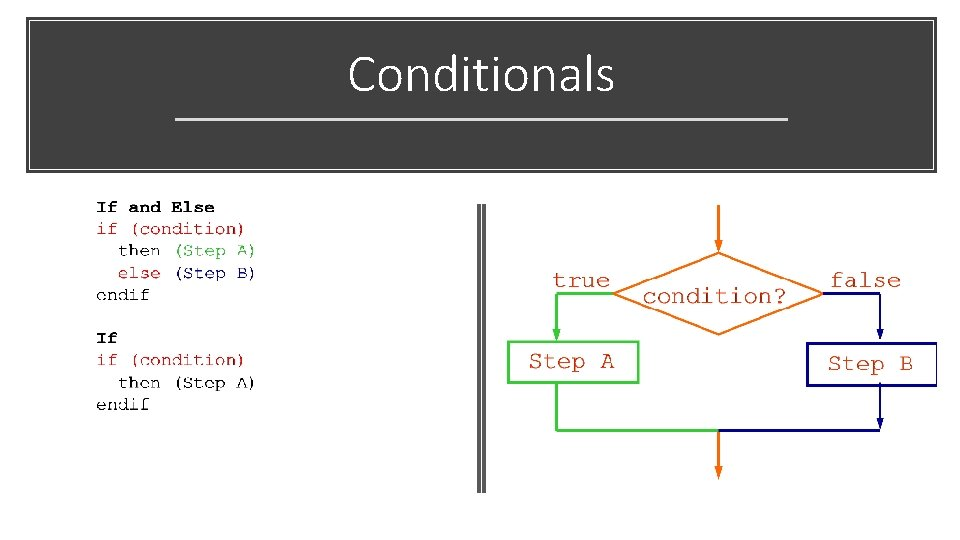ConditionalsLet mark be the total-mark obtained if (mark < 40) Conditionals • then (print “Student fail”) • else (print “Student pass”) endif …read in mark (*from a list*) if (mark < 40) then (Grade “F”) Conditionals • else if (mark < 50) then (Grade “D”) • else if (mark < 60) then (Grade “C”) • else if (mark < 70) then (Grade “B”) • else if (mark < 80) then (Grade “A”); endif print “Student grade is”, Grade …Implement the pseudocode in R Conditionals Try to be as detailed as you can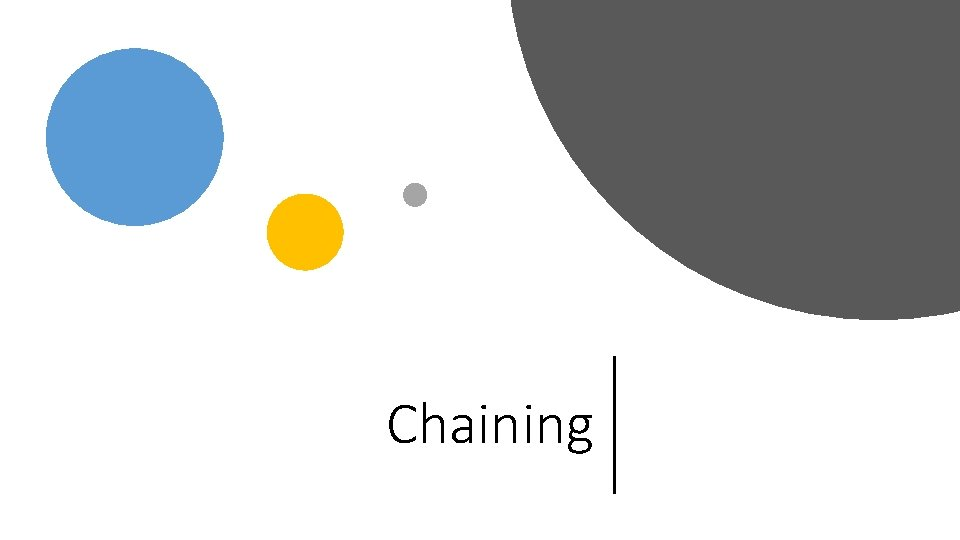ChainingYou will realize that IF conditions are basically testing for a logical outcome Logical/Boolean can be chained together using AND and OR operators AND and OR are also known as Boolean operators Syntax alert! • AND in R is represented by ‘&&’ • OR in R is represented by ‘||’The AND operator • AND returns TRUE only if both conditions are also simultaneously fulfilled (i. e. , returns as TRUE) • You can also understand this by looking at the Venn diagram below where rivers AND salinity only returns the intersection between them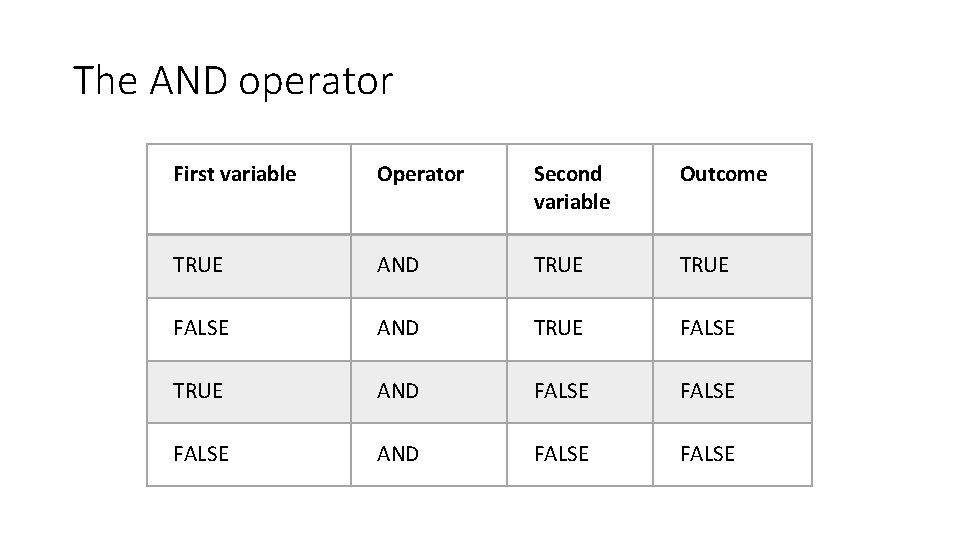The AND operator First variable Operator Second variable Outcome TRUE AND TRUE FALSE TRUE AND FALSE AND FALSE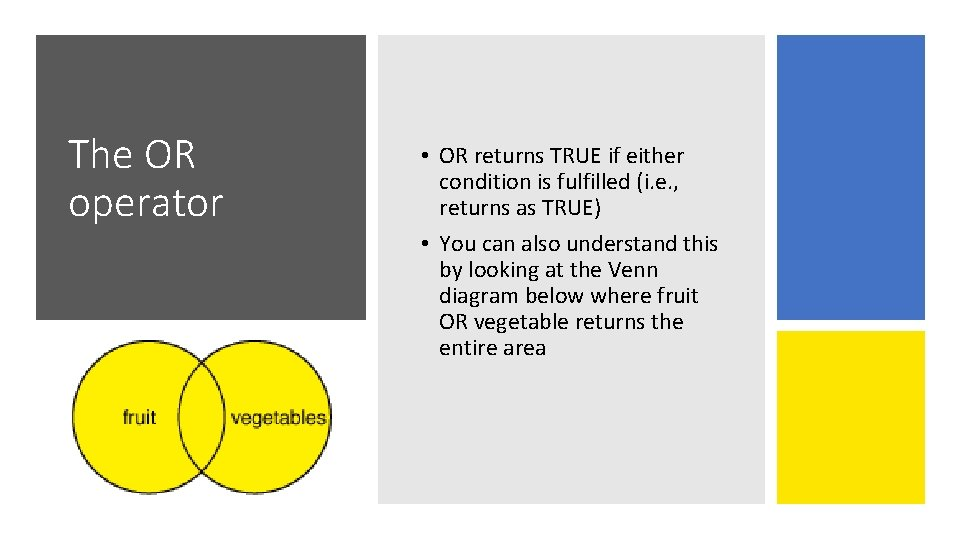The OR operator • OR returns TRUE if either condition is fulfilled (i. e. , returns as TRUE) • You can also understand this by looking at the Venn diagram below where fruit OR vegetable returns the entire area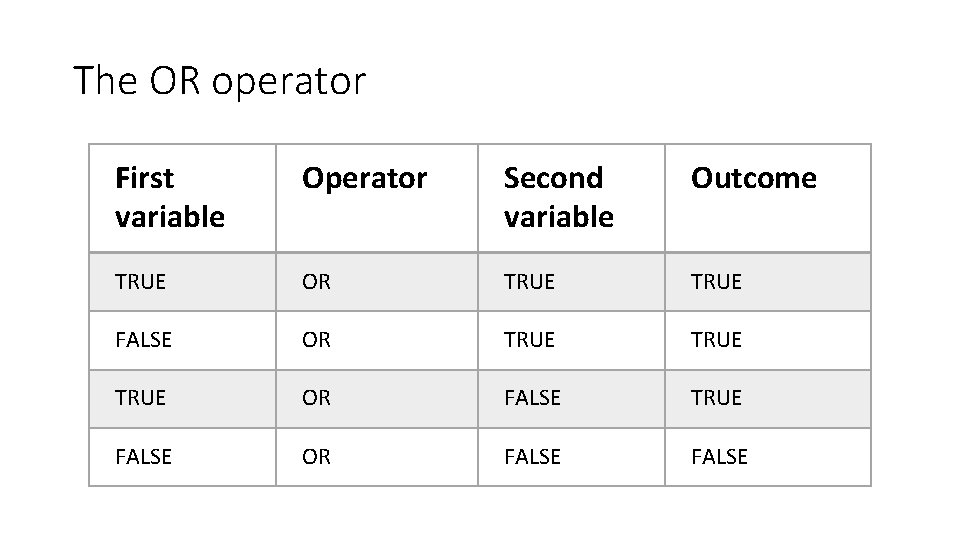The OR operator First variable Operator Second variable Outcome TRUE OR TRUE FALSE OR TRUE OR FALSE TRUE FALSE OR FALSE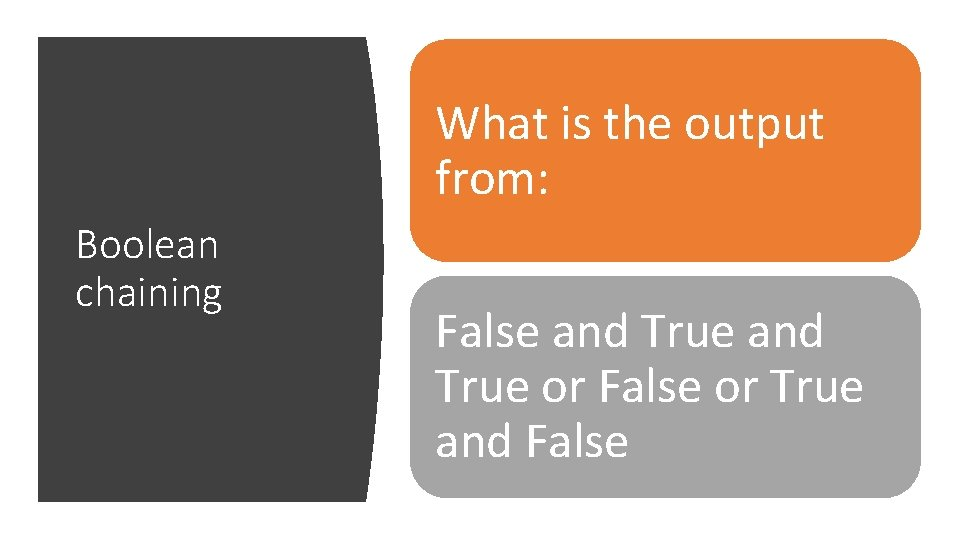What is the output from: Boolean chaining False and True or False or True and False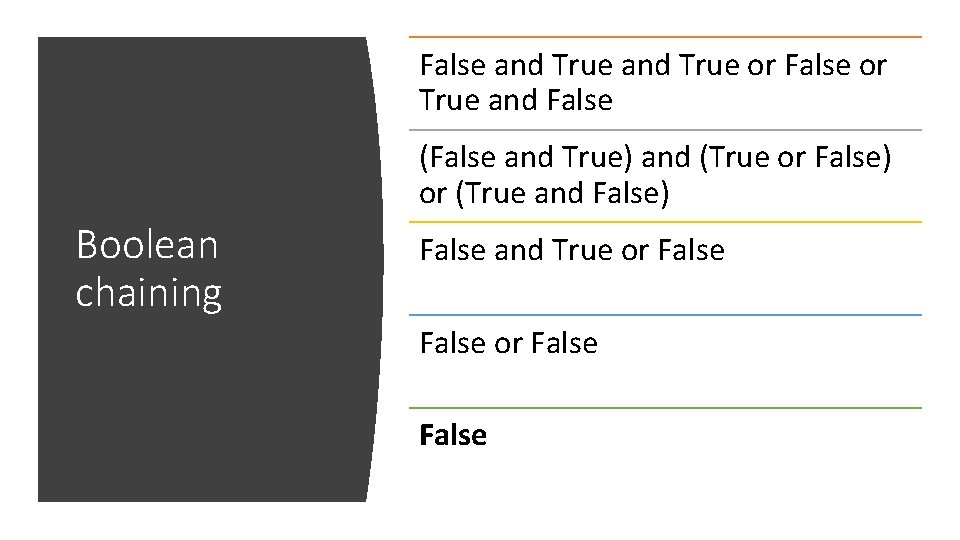False and True or False or True and False (False and True) and (True or False) or (True and False) Boolean chaining False and True or FalseWhat is the practical significance of chaining Boolean? When you have multiple conditions that need to be fulfilled in some manner Like finding an ideal marriage partner! Which denotes a stronger requirement? Must be Kind Must be Smart Must be Rich Must be Asian Kind AND Smart AND Rich AND Asian? Kind AND SMART OR Rich OR Asian?You can also do chaining with numerical comparisons What is the practical significance of chaining Boolean? The syntax for AND in R is double or single ampersand (&& or &) measured_BP <- 130 Try: #A good blood pressure needs to be below 140 and also above 90 (90 < measured_BP) && (measured_BP < 140) #This returns as TRUE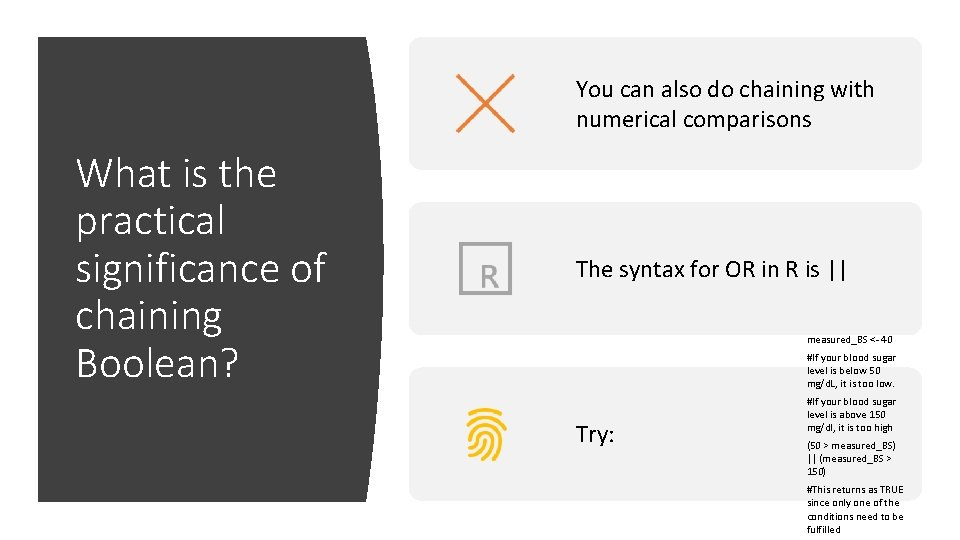You can also do chaining with numerical comparisons What is the practical significance of chaining Boolean? The syntax for OR in R is || measured_BS <- 40 #If your blood sugar level is below 50 mg/d. L, it is too low. Try: #If your blood sugar level is above 150 mg/dl, it is too high (50 > measured_BS) || (measured_BS > 150) #This returns as TRUE since only one of the conditions need to be fulfilled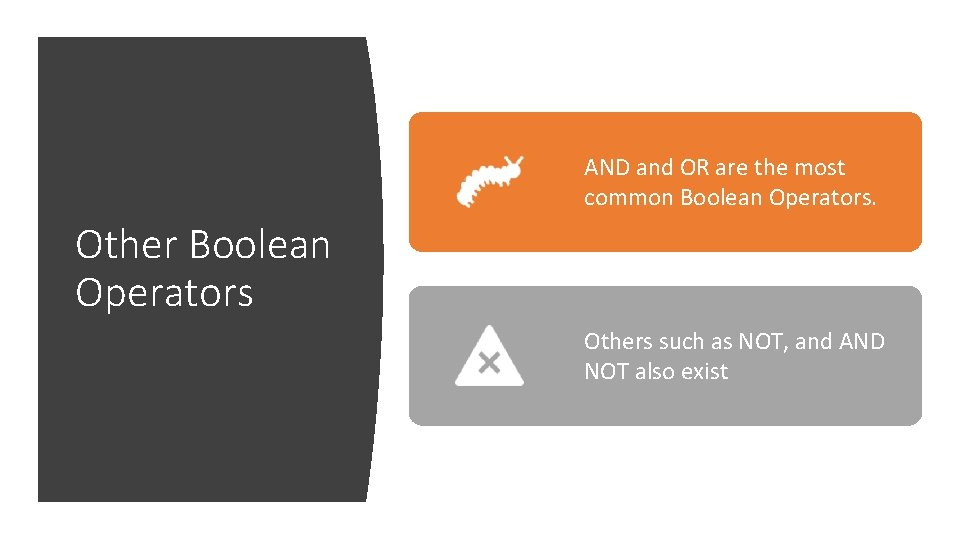AND and OR are the most common Boolean Operators. Other Boolean Operators Others such as NOT, and AND NOT also exist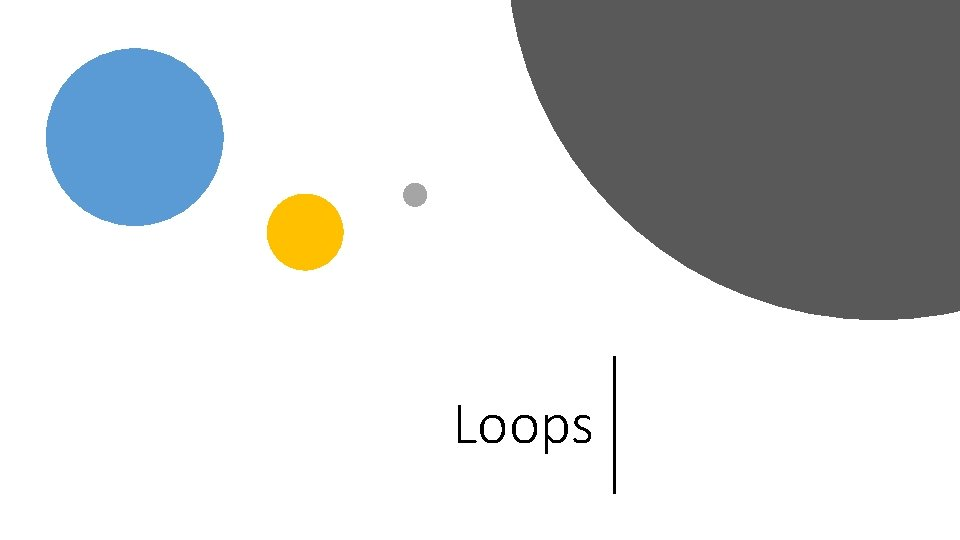Loops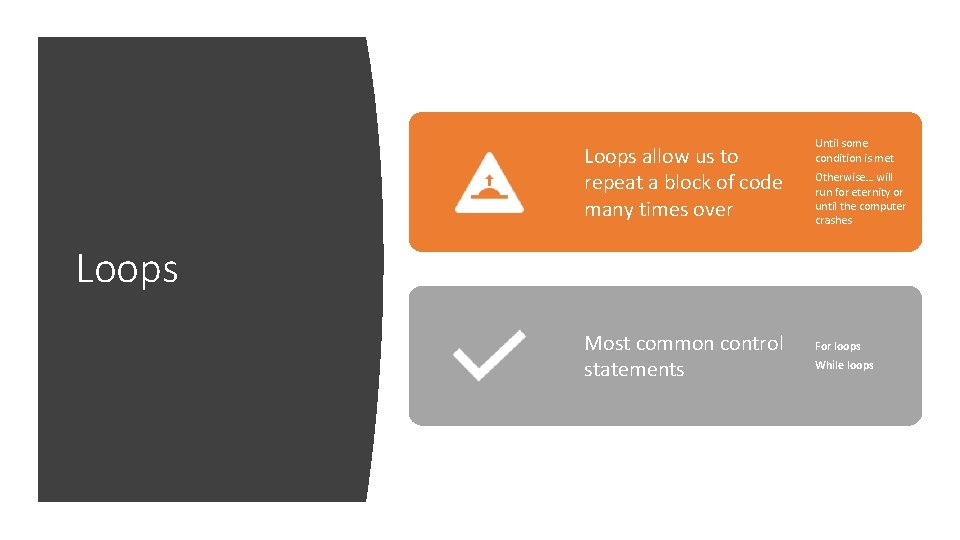Loops allow us to repeat a block of code many times over Until some condition is met Otherwise… will run for eternity or until the computer crashes Loops Most common control statements For loops While loopsConsider the following problem: I want to add the following numbers from 1, 2, 3, 4, … 10. Why do we want to loop? One way of doing this is to manually write 1 + 2 + … + 10 But what if I want to add from 1 to 100? This would be very inefficient One elegant solution to resolve this “verbose” issue is to write a loop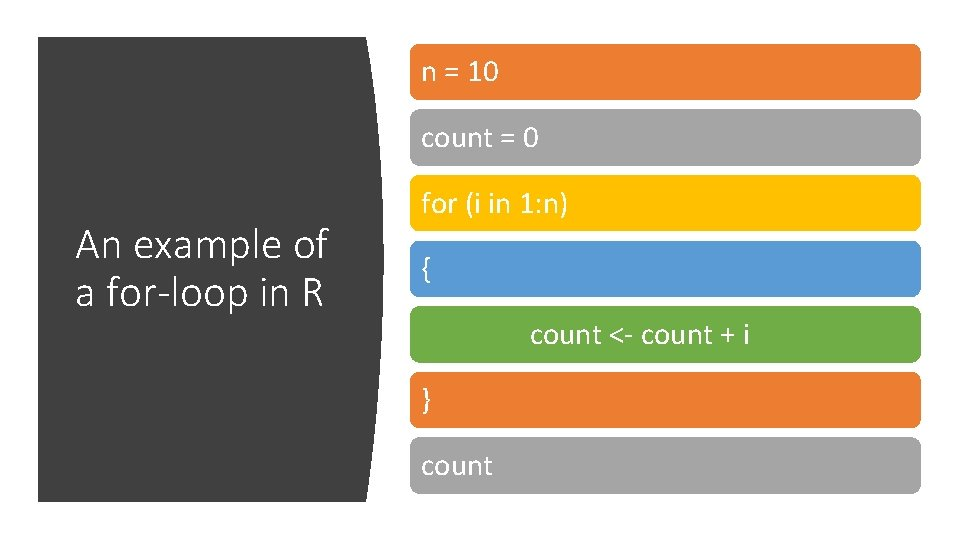n = 10 count = 0 An example of a for-loop in R for (i in 1: n) { count <- count + i } countThere are 4 main components in a loop Initialize (set up a control variable that controls the loop) Test (do we continue running the loop? ) Loop body (part that needs to be repeated) Update (update the control variable)Control statements (for-loop)n = 10 i=1 count = 0 An example of a while loop in R while (i <= n) { count <- count + i i <- i + 1 } count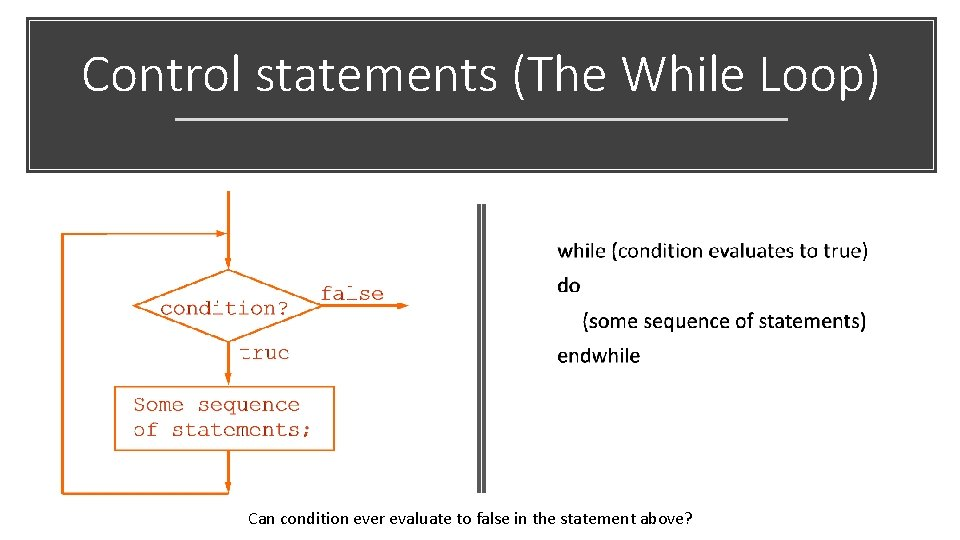Control statements (The While Loop) Can condition ever evaluate to false in the statement above?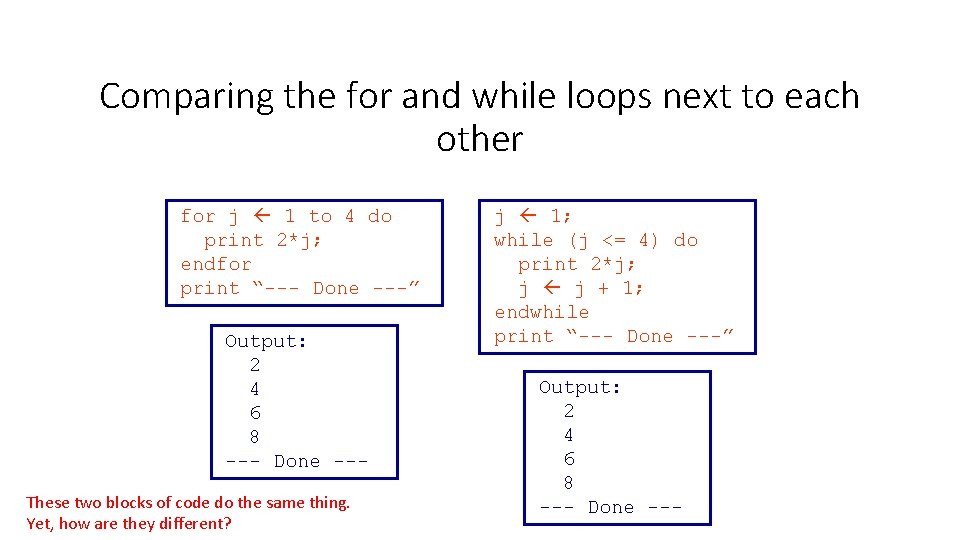Comparing the for and while loops next to each other for j 1 to 4 do print 2*j; endfor print “--- Done ---” Output: 2 4 6 8 --- Done --These two blocks of code do the same thing. Yet, how are they different? j 1; while (j <= 4) do print 2*j; j j + 1; endwhile print “--- Done ---” Output: 2 4 6 8 --- Done ---R Data structuresData structures in R • Data types can be assembled into larger and more complex entities called data structures • R offers a wide variety of data structures for satisfying different task requirementsData structures in R Factor A A B B -It is 1 column or row -Contains “level” data which describes “levels” of classification e. g. class label A or B List -a collection of entities with different lengths - multiple types - Multiple data structures (vectors, matrices and data frames)End of Segment Let’s take a break